A stone dropped from a building of height h and it reaches after t seconds on earth. From the same building if two stones are thrown (one upwards and other downwards) with the same velocity u and they reach the earth surface after t1 and t2 seconds respectively, then

(1) $t={t}_{1}-{t}_{2}$

(2) $t=\frac{{t}_{1}+{t}_{2}}{2}$

(3) $t=\sqrt{{t}_{1}{t}_{2}}$

(4) $t={t}_{1}^{2}{t}_{2}^{2}$

Concept Questions :-

Uniformly accelerated motion
High Yielding Test Series + Question Bank - NEET 2020

Difficulty Level:

A ball is projected upwards from a height h above the surface of the earth with velocity v. The time at which the ball strikes the ground is

(1) $\frac{v}{g}+\frac{2hg}{\sqrt{2}}$

(2) $\frac{v}{g}\left[1-\sqrt{1+\frac{2h}{g}}\right]$

(3) $\frac{v}{g}\left[1+\sqrt{1+\frac{2gh}{{v}^{2}}}\right]$

(4) $\frac{v}{g}\left[1+\sqrt{{v}^{2}+\frac{2g}{h}}\right]$

Concept Questions :-

Uniformly accelerated motion
High Yielding Test Series + Question Bank - NEET 2020

Difficulty Level:

A particle is dropped vertically from rest from a height. The time taken by it to fall through successive distances of 1 m each will then be

(1) All equal, being equal to $\sqrt{2/g}$ second

(2) In the ratio of the square roots of the integers 1, 2, 3.....

(3) In the ratio of the difference in the square roots of the integers i.e. $\sqrt{1},\text{\hspace{0.17em}}\left(\sqrt{2}-\sqrt{1}\right),\text{\hspace{0.17em}}\left(\sqrt{3}-\sqrt{2}\right),\text{\hspace{0.17em}}\left(\sqrt{4}-\sqrt{3}\right)$ ....

(4) In the ratio of the reciprocal of the square roots of the integers i.e.,. $\frac{1}{\sqrt{1}},\text{\hspace{0.17em}}\frac{1}{\sqrt{2}},\frac{1}{\sqrt{3}},\text{\hspace{0.17em}}\frac{1}{\sqrt{4}}$

High Yielding Test Series + Question Bank - NEET 2020

Difficulty Level:

A man throws balls with the same speed vertically upwards one after the other at an interval of 2 seconds. What should be the speed of the throw so that more than two balls are in the sky at any time (Given $g=9.8m/{s}^{2}\right)$

(1) At least 0.8 m/s

(2) Any speed less than 19.6 m/s

(3) Only with speed 19.6 m/s

(4) More than 19.6 m/s

Concept Questions :-

Acceleration
High Yielding Test Series + Question Bank - NEET 2020

Difficulty Level:

If a ball is thrown vertically upwards with speed u, the distance covered during the last t seconds of its ascent is

(1) $\frac{1}{2}g{t}^{2}$

(2) $ut-\frac{1}{2}g{t}^{2}$

(3) $\left(u-gt\right)t$

(4) $ut$

Concept Questions :-

Uniformly accelerated motion
High Yielding Test Series + Question Bank - NEET 2020

Difficulty Level:

The variation of velocity of a particle with time moving along a straight line is illustrated in the following figure. The distance travelled by the particle in four seconds is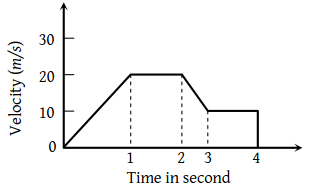1. 60 m

2. 55 m

3. 25 m

4. 30 m

Concept Questions :-

Graphs
High Yielding Test Series + Question Bank - NEET 2020

Difficulty Level:

The displacement of a particle as a function of time is shown in the figure. The figure shows that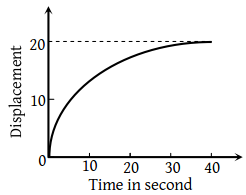1. The particle starts with certain velocity but the motion is retarded and finally the particle stops

2. The velocity of the particle is constant throughout

3. The acceleration of the particle is constant throughout.

4. The particle starts with constant velocity, then motion is accelerated and finally the particle moves with another constant velocity

Concept Questions :-

Graphs
High Yielding Test Series + Question Bank - NEET 2020

Difficulty Level:

A ball is thrown vertically upwards. Which of the following graph/graphs represent velocity-time graph of the ball during its flight (air resistance is neglected)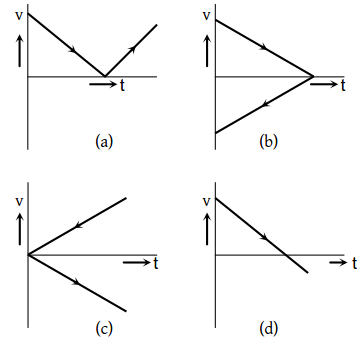1. a

2. b

3. c

4. d

Concept Questions :-

Uniformly accelerated motion
High Yielding Test Series + Question Bank - NEET 2020

Difficulty Level:

The graph between the displacement x and time t for a particle moving in a straight line is shown in figure. During the interval $OA,\text{\hspace{0.17em}}AB,\text{\hspace{0.17em}}BC$ and CD, the acceleration of the particle is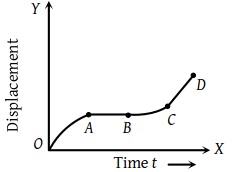OA AB BC CD (1) + 0 + + (2) – 0 + 0 (3) + 0 – + (4) – 0 – 0

Concept Questions :-

Graphs
High Yielding Test Series + Question Bank - NEET 2020

Difficulty Level:

The vt graph of a moving object is given in figure. The maximum acceleration is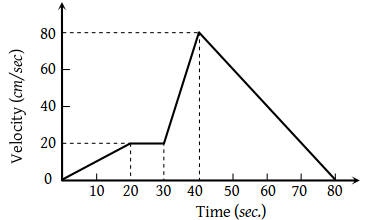1. $1cm/se{c}^{2}$

2. $2cm/se{c}^{2}$

3. $3\text{\hspace{0.17em}\hspace{0.17em}}cm/se{c}^{2}$

4. $6\text{\hspace{0.17em}\hspace{0.17em}}cm/se{c}^{2}$

Concept Questions :-

Graphs